# Learning Electronics

Learn to build electronic circuits

# Energy, Work, and Power

### Question 1:

Define the following terms: energy, work, and power.
Work is the exertion of a force over a distance. Energy is the capacity to perform work. Power is the rate of work performed per unit time.

Notes:
Students may find a basic physics text helpful in obtaining these definitions. "Work" is a difficult concept to precisely define, especially for students unfamiliar with basic physics. Technically, it is the vector dot-product of force and displacement, meaning that work equals force times distance only if the force and distance vectors are precisely parallel to each other. In other words, if I carry a 10 kg mass (lifting up against the tug of gravity) while walking parallel to the ground (not going up or down), the force and displacement vectors are perpendicular to each other, and the work I do in carrying the mass is zero. It is only if my force is directed precisely the same direction as my motion that all of my effort is translated into work.

### Question 2:

For a given amount of electric current, which resistor will dissipate the greatest amount of power: a small value (low-resistance) resistor, or a high value (high-resistance) resistor? Explain your answer.
A resistor with a high resistance rating (many öhms" of resistance) will dissipate more heat power than a lower-valued resistor, given the same amount of electric current through it.

Notes:
This question is designed to make students think qualitatively about the relationship between current, resistance, and power. I have found that qualitative (non-numeric) analysis is often more challenging than asking students to calculate answers quantitatively (with numbers). Often, simple math is a kind of barrier behind which students seek refuge from true understanding of a topic. In other words, it is easier to punch keys on a calculator (or even perform calculations with paper and pencil) than to really think about the inter-relationships of variables in a physical problem. Yet, a qualitative understanding of electrical systems is vital to fast and efficient troubleshooting.

### Question 3:

Plot the relationship between power and current for a 2 W resistor on this graph: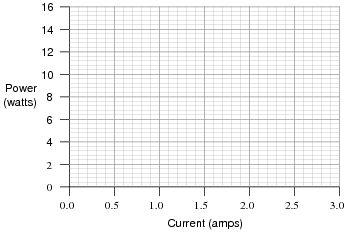What pattern do you see represented by plot? How does this compare with the graphical relationship between voltage and current for a resistor?
The more current through the resistor, the more power dissipated. However, this is not a linear function!

Notes:
Students need to become comfortable with graphs, and creating their own simple graphs is an excellent way to develop this understanding. A graphical representation of the Ohm's Law (actually, Joule's Law) power function allows students another "view" of the concept.
If students have access to either a graphing calculator or computer software capable of drawing 2-dimensional graphs, encourage them to plot the functions using these technological resources.

### Question 4:

Shown here is a schematic diagram for a simple battery-powered flashlight:What could be modified about the circuit or its components to make the flashlight produce more light when turned on?
Somehow, the power dissipated by the light bulb must be increased. Perhaps the most obvious way to increase power dissipation is to use a battery with a greater voltage output, thus giving greater bulb current and greater power. However, this is not the only option! Think of another way the flashlight's output may be increased.

Notes:
The öbvious" solution is a direct application of Ohm's Law. Other solutions may not be so direct, but they will all relate back to Ohm's Law somehow.

### Question 5:

There are two basic Ohm's Law equations: one relating voltage, current, and resistance; and the other relating voltage, current, and power (the latter equation is sometimes known as Joule's Law rather than Ohm's Law):

 E = I R

 P = I E

In electronics textbooks and reference books, you will find twelve different variations of these two equations, one solving for each variable in terms of a unique pair of two other variables. However, you need not memorize all twelve equations if you have the ability to algebraically manipulate the two simple equations shown above.
Demonstrate how algebra is used to derive the ten öther" forms of the two Ohm's Law / Joule's Law equations shown here.
I won't show you how to do the algebraic manipulations, but I will show you the ten other equations. First, those equations that may be derived strictly from E = I R:

 I = E R

 R = E I

Next, those equations that may be derived strictly from P = I E:

 I = P E

 E = P I

Next, those equations that may be derived by using algebraic substitution between the original two equations given in the question:

 P = I2 R

 P = E2 R

And finally, those equations which may be derived from manipulating the last two power equations:

 R = P I2

I =

 P R

 E = � P R

 R = E2 P

Notes:
Algebra is an extremely important tool in many technical fields. One nice thing about the study of electronics is that it provides a relatively simple context in which fundamental algebraic principles may be learned (or at least illuminated).
The same may be said for calculus concepts as well: basic principles of derivative and integral (with respect to time) may be easily applied to capacitor and inductor circuits, providing students with an accessible context in which these otherwise abstract concepts may be grasped. But calculus is a topic for later worksheet questions . . .

### Question 6:

Suppose someone mechanically couples an electric motor to an electric generator, then electrically couples the two devices together in an effort to make a perpetual-motion machine: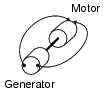Why won't this assembly spin forever, once started?
This will not work because neither the motor nor the generator is 100% efficient.

Notes:
The easy answer to this question is "the Law of Conservation of Energy (or the Second Law of Thermodynamics) forbids it," but citing such a "Law" really doesn't explain why perpetual motion machines are doomed to failure. It is important for students to realize that reality is not bound to the physical "Laws" scientists set; rather, what we call "Laws" are actually just descriptions of regularities seen in nature. It is important to emphasize critical thinking in a question like this, for it is no more intellectually mature to deny the possibility of an event based on dogmatic adherence to a Law than it is to naively believe that anything is possible.

### Question 7:

Suppose a mass is connected to a winch by means of a cable, and a person turns the winch drum to raise the mass off the ground: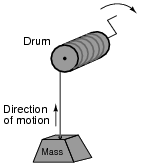A physicist would likely look at this scenario as an example of energy exchange: the person turning the drum is expending energy, which in turn is being stored in the mass in potential form.
Suppose now that the person stops turning the drum and instead engages a brake mechanism on the drum so that it reverses rotation and slowly allows the mass to return to ground level. Once again, a physicist would view this scenario as an exchange of energy: the mass is now releasing energy, while the brake mechanism is converting that released energy into heat:In each of the above scenarios, draw arrows depicting directions of two forces: the force that the mass exerts on the drum, and the force that the drum exerts on the mass. Compare these force directions with the direction of motion in each scenario, and explain how these directions relate to the mass and drum alternately acting as energy source and energy load.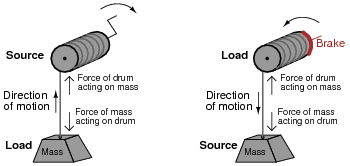Follow-up question: although it may not be obvious, this question closely relates to the exchange of energy between components in electrical circuits! Explain this analogy.

Notes:
Students typically find the concept of energy flow confusing with regard to electrical components. I try to make this concept clearer by using mechanical analogies, in which force and motion act as analog quantities to voltage and current (or visa-versa).

### Question 8:

Draw the direction of current in this circuit, and also identify the polarity of the voltage across the battery and across the resistor. Then, compare the battery's polarity with the direction of current through it, and the resistor's polarity with the direction of current through it.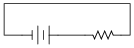What do you notice about the relationship between voltage polarity and current direction for these two different types of components? Identify the fundamental distinction between these two components that causes them to behave differently.
Here I show the answer in two different forms: current shown as electron flow (left) and current shown as conventional flow (right).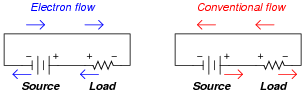Whichever notation you choose to follow in your analysis of circuits, the understanding should be the same: the reason voltage polarities across the resistor and battery differ despite the same direction of current through both is the flow of power. The battery acts as a source, while the resistor acts as a load.

Notes:
This type of distinction is very important in the study of physics as well, where one must determine whether a mechanical system is doing work or whether work is being done on it. A clear understanding of the relationship between voltage polarity and current direction for sources and loads is very important for students to have before they study reactive devices such as inductors and capacitors!

### Question 9:

When an electric current travels through an electrical resistance, not only will there be a voltage "drop" across that resistance (V = IR), but there will also be energy dissipated by that resistance. We describe the rate of energy dissipation over time as power (P), and we express power in the unit of the watt (W).
Write the equation relating power to voltage and current, for an electrical resistance. Also, describe what physical form this dissipated energy usually takes.

 P = IV

Energy dissipated by an electrical resistance is usually manifest as heat, although sometimes it is also in the form of light.

Notes:
Most people, even if they have no prior experience with electric circuit analysis, have some sense of what a "Watt" is, because so many consumer-grade appliances are rated in watts (light bulbs, heaters, blow driers, etc.). Use these examples as context for your discussion on electric power.

### Question 10:

What would happen if a wire having no resistance at all (0 W) were connected directly across the terminals of a 6-volt battery? How much power would be dissipated, according to Joule's Law?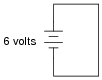Suppose I short-circuited a 6-volt battery in the manner just described and found that the wire used to make the short-circuit gets warm after just a few seconds of carrying this current. Does this data agree or disagree with your predictions?
Calculations based on power equations would suggest either zero watts of power dissipated or infinite power dissipation, depending on which equation you chose to calculate power with. Yet, the experiment described yields a power dissipation that is neither zero nor infinite.
If you think that the wire used in the experiment is not resistance-less (i.e. it does have resistance), and that this accounts for the disparity between the predicted and measured amounts of current, you are partially correct. Realistically, a small piece of wire such as that used in the experiment will have a few tenths of an ohm of resistance. However, if you re-calculate power with a wire resistance of 0.1 W, you will still find a large disparity between your prediction and the actual measured power in this short-circuit.
What is going on here?

Notes:
Remind students that short-circuit testing of electrical power sources can be dangerous. A student of mine once stuffed a 6-volt "lantern" battery in his tool pouch, only to have it discharge smoke an hour later, after the battery terminals had been shorted together by the metal handle of a wrench!

### Question 11:

How much electrical power is being dissipated by the light bulb in this circuit?In this circuit, identify which component is the source and which is the load.
6.48 watts
Here, the battery is the source and the light bulb is the load.

Notes:
The solution to this problem simply requires the application of Ohm's Law. As for the question of source versus load, ask your students to define each of these words in terms of energy transfer.

### Question 12:

Calculate the amount of power dissipated by this electric heating element, if the generator's output voltage is 110 volts and the heater's resistance is 2.5 ohms: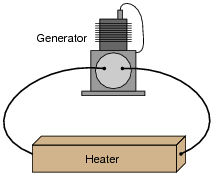Now, calculate the power dissipated by the same heater if the generator's output voltage is doubled.
4,840 watts at 110 volts; 19,360 watts at 220 volts.

Notes:
Many students will mistakenly calculate 9,680 watts as the power dissipation at 220 volts. However, power dissipation does not increase linearly with increases in voltage!

### Question 13:

A DC electric motor transforms 1.75 kW of electrical power into mechanical form. If the motor's operating voltage is 300 volts, how much current does it "draw" when operating at full load (full power output)?
Also calculate the motor's output power in units of horsepower, assuming 100% efficiency.
Motor current = 5.833 amps. Motor power = 2.346 horsepower.

Notes:
To answer both parts of this question, students will have to apply Ohm's (Joule's) Law to calculate power from figures of voltage and current, and they will also have to research the conversion factor between watts and horsepower.

### Question 14:

Two construction workers, standing on the roof of a building, hoist identical buckets of tools to the top by pulling upward on long ropes. The weight of the tools and buckets are the same for each worker, as is the distance each bucket is hoisted. However, one worker hoists their bucket from the ground to the roof in one-third the time that it takes the other worker. Compare the efforts of the two workers in terms of energy and power.
Both workers perform the same amount of work, and expend the same amount of energy in doing so. However, the worker who hoists the bucket up in only one-third the time performed that work with three times as much power as the slower worker.

Notes:
It is a common misconception to equate energy with power. Energy, technically, is a measure that is irrespective of time. Power, on the other hand, implicitly references the time taken to do a certain amount of work.